# The MI Procedure

### Multiple Imputation with Pattern-Mixture Models

For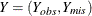, the joint distribution of `Y` andcan be expressed as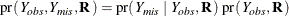Under the MAR assumption,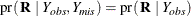and it can be shown that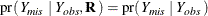That is,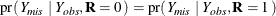Thus the posterior distribution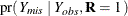can be used to create imputations for missing data.

Under the MNAR assumption, each pattern that has missing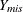values might have a different distribution than the corresponding pattern that has observedvalues. For example, in a clinical trial, suppose the data set contains an indicator variable `Trt`, with a value of 1 for patients in the treatment group and a value of 0 for patients in the placebo control group, a variable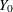for the baseline efficacy score, and a variable `Y` for the efficacy score at a follow-up visit. Assume that `Trt` andare fully observed and `Y` is not fully observed. The indicator variableis 0 or 1, depending on whether `Y` is missing or observed.

Then, under the MAR assumption,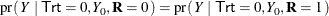and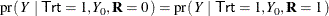Under the MNAR assumption,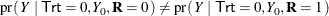or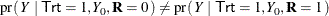Thus, under MNAR, missing `Y` values in the treatment group can be imputed from a posterior distribution generated from observations in the control group, and the imputed values can be adjusted to reflect the systematic difference between the distributions for missing and observed `Y` values.

Multiple imputation inference, under either the MAR or MNAR assumption, involves three distinct phases:

1. The missing data are filled in m times to generate m complete data sets.

2. The m complete data sets are analyzed by using other SAS procedures.

3. The results from the m complete data sets are combined for the inference.

For sensitivity analysis, you must specify the MNAR statement together with a MONOTONE statement or an FCS statement. When you specify a MONOTONE statement, the variables that have missing values are imputed sequentially in each imputation. When you specify an FCS statement, each imputation is carried out in two phases: the preliminary filled-in phase, followed by the imputation phase. The variables that have missing values are imputed sequentially for a number of burn-in iterations before the imputation.

Under the MNAR assumption, the following steps are used to impute missing values for each imputed variable in each imputation (when you specify a MONOTONE statement) or in each iteration (when you specify an FCS statement):

1. For each imputed variable, a conditional model, such as a regression model for continuous variables, is fitted using either all applicable observations or a specified subset of observations.

2. A new model is simulated from the posterior predictive distribution of the fitted model.

3. Missing values of the variable are imputed based on the new model, and the imputed values for a specified subset of observations can be adjusted using specified shift and scale parameters.

The next two sections provide details for specifying subsets of observations for imputation models and for adjusting imputed values.